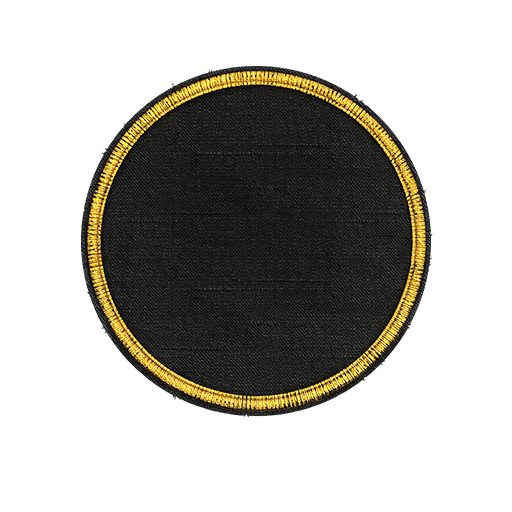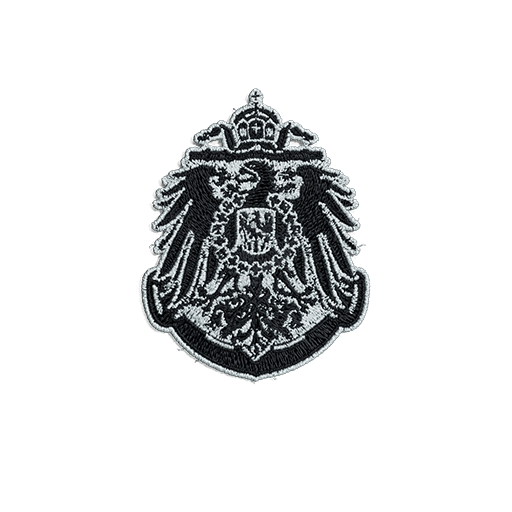Kuramitsu

Beta Testers

653

[WOLF2]

Community Reputation

430 Excellent

• Rank
Warrant Officer
• InsigniaProfile Information

• Gender
Not Telling
• Location
• Interests
German Anime Waifus

Recent Profile Visitors

2,868 profile views

• MarksEyes

1. What is it? I see this was posted 3hrs ago and I'm stuck at work.
2. Thank you, I mainly wanted to post this about the coupon and how you actually use it. Saw some players having issues with it.
3. Today is my B-Day and I got to use my coupon to get some nice goodies. I bought Agir and use my 100% dubloons to get 19300 back the use my dubloons coupon I've been saving ingame to get Z-44 at a discount which left me with enough to get 3 black Friday containers which had 100,000 captains xp, 14days of premium, 21 black camo. FYI be sure to check your email that is connected to your account for the birthday notice (mine was in the junk mail) then login to the game so it will activate the coupon (plus it gives you 2 SCs and premium time). This way you don't accidentally buy something without the coupon active yet. Once that's all done you can go to your coupon section and click on it and it will show you what you can buy that will refund you the 100% gold back. Be mindful that it only works on one item.
• 6 replies
• 4
••4. Open mine up got 21 camo
5. All the German CVs need a buff
• 198 replies
• 4
•••6. 𝑦=−21 13 1 Distribute −5𝑦+3=2(4𝑦+12) -5y+3={\color{#c92786}{2(4y+12)}}−5y+3=2(4y+12) −5𝑦+3=8𝑦+24 -5y+3={\color{#c92786}{8y+24}}−5y+3=8y+24 2 Subtract 3 33 from both sides of the equation −5𝑦+3=8𝑦+24 -5y+3=8y+24−5y+3=8y+24 −5𝑦+3−3=8𝑦+24−3 -5y+3{\color{#c92786}{-3}}=8y+24{\color{#c92786}{-3}}−5y+3−3=8y+24−3 3 Simplify Subtract the numbers Subtract the numbers −5𝑦=8𝑦+21 -5y=8y+21−5y=8y+21 4 Subtract 8𝑦 8y8y from both sides of the equation −5𝑦=8𝑦+21 -5y=8y+21−5y=8y+21 −5𝑦−8𝑦=8𝑦+21−8𝑦 -5y{\color{#c92786}{-8y}}=8y+21{\color{#c92786}{-8y}}−5y−8y=8y+21−8y 5 Simplify Combine like terms Combine like terms −13𝑦=21 -13y=21−13y=21 6 Divide both sides of the equation by the same term −13𝑦=21 -13y=21−13y=21 −13𝑦−13=21−13 \frac{-13y}{{\color{#c92786}{-13}}}=\frac{21}{{\color{#c92786}{-13}}}−13−13y=−1321 7 Simplify Cancel terms that are in both the numerator and denominator Divide the numbers 𝑦=−2113 y=-\frac{21}{13}y=−1321 Show less Solution 𝑦=−2113
7. If it's not German then it's not worth it.
• 63 replies
• 2
••×

• Calendar